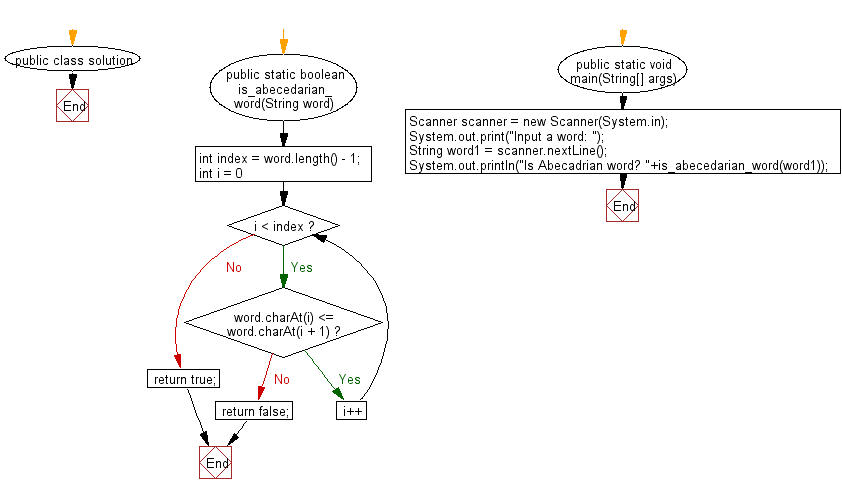# Java Exercises: Check if each letter of a given word is less than the one before it

## Java Basic: Exercise-248 with Solution

From Wikipedia, An abecedarium (or abecedary) is an inscription consisting of the letters of an alphabet, almost always listed in order. Typically, abecedaria (or abecedaries) are practice exercises.
Write a Java program to check if each letter of a given word (Abecadrian word) is less than the one before it.

Sample Solution:

Java Code:

``````import java.util.*;
public class solution {
public static boolean is_abecedarian_word(String word) {
int index = word.length() - 1;

for (int i = 0; i < index; i++) {

if (word.charAt(i) <= word.charAt(i + 1)) {
}

else {
return false;
}
}
return true;
}

public static void main(String[] args) {
Scanner scanner = new Scanner(System.in);
System.out.print("Input a word: ");
String word1 = scanner.nextLine();
}
}
```
```

Sample Output:

```Input a word:  ABCD
```

Flowchart:Java Code Editor:

What is the difficulty level of this exercise?

﻿

## Java: Tips of the Day

Parsing dates:

```import java.io.*;
import java.util.*;
import java.text.*;

String s = "2001/09/23 14:39";

SimpleDateFormat formatter = new SimpleDateFormat ("yyyy/MM/dd H:mm");
Date d = formatter.parse(s, new ParsePosition(0));
```